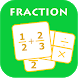# Easy Fraction CalculatorFree App

Rated 4.25/5 (4) —  Free Android application by Recommended Mobile Apps

Simple Fraction Calculator is the best application and easiest way to deal with fraction problems. It can help you checking math homework, working on craft or construction projects.
- Add, substract, multiply, and divide fractions. Fraction operator couldn't been easier.
- Show fraction calculations in crisp, clear, elegant type.
- Every result you got automatically reduced to its simplest form (simplify fraction).
- Every result is also shown in decimal.
- Simple and easy user interface lets you type fast!
So if you want to check your math homework, it's easy and takes just seconds.

Hightlight features:
- Mixed fraction calculator - add, substract, mutiply, divide fraction
- Reduce fraction to its simplest form
- Fraction to decimal convertor
- Decimal to fraction convertor

Let Simple Fraction Calculator turn your phone or table into everyday helping hand.
This is an ad supported version, you may see ads while using it but it's free application, free fraction calculator.
If you find it useful please rate 5 star. Thank you for your support.

Free
downl.
Android
4.0+
For everyone
Android app

## What are users saying about Easy Fraction Calculator

Z70%
by Z####:

Its great. It help my child a lot with her learning.

Z70%
by Z####:

Nice and accurate

RATING
4.35
4 users

5

4

3

2

1